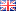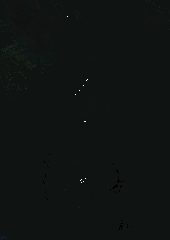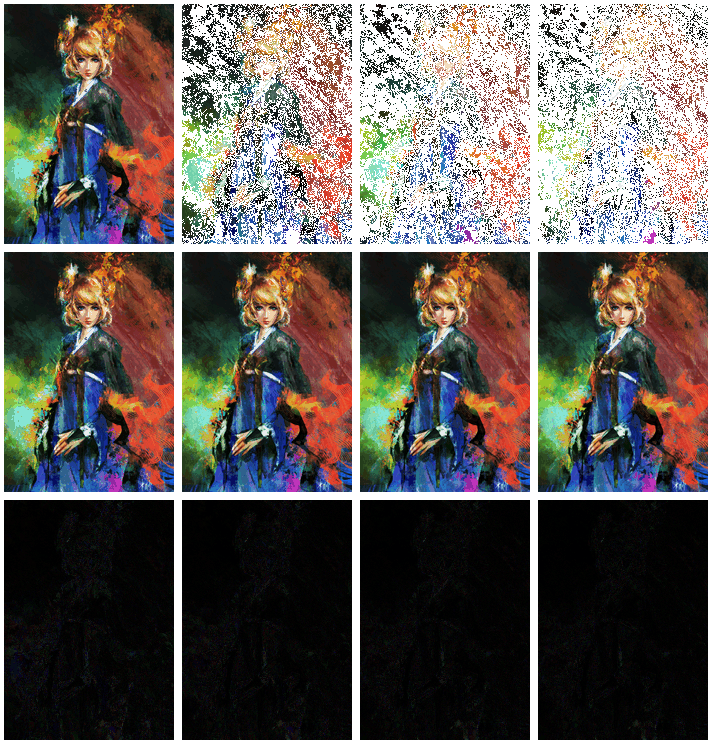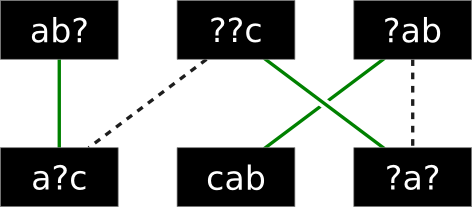## High-Color GIF ImagesIn the nineties the prevalent image format used on the world wide web was the GIF file format (full spec), which is reasonably efficient, widely portable, and supports animation and transparency. Mainly inspired by concerns over patent claims by Unisys on the LZW compression algorithm used to create GIF files, proponents of open web standards designed the PNG file format (based on the DEFLATE compression algorithm, which is believed to be unencumbered by patents) as a replacement. PNG does have several advantages over GIF, but some of the PNG-advocacy from the time was misleading.

One common reason suggested to prefer PNG over GIF, was that GIF files are limited to using 256-color palettes, and therefore unsuitable for rendering color images faithfully. It turns out this isn't quite true! GIF files can, in fact, contain an arbitrary number of colors, and in this post I will show how such high-color GIF images can be constructed that are quite suitable for web use.

#### Technical details

Althought it's true that GIF files are limited to an 8-bit pixel format (each pixel value indexing a table of at most 256 colors) a single GIF image may contain multiple graphic rendering blocks, each of which may include its own local color table of 256 colors drawn from a 24-bit color space.

Since a single image can display multiple graphic rendering blocks at once (either by rendering separate blocks into separate regions of the canvas, or by using transparency to overlap blocks without overwriting all previous pixels) the final image can contain an arbitrary number of different colors.

An easy approach to generating high-color GIF images is to partition the set of colors used over multiple frames which are then rendered on top of each other. The first frame contains the pixels with the 256 most-commonly used colors, while each next frame adds 255 new colors (reserving one palette index for transparency).170x240 pixels, 36330 colors, 24-bit PNG, 88 KB 170x240 pixels, 36330 colors, 143 frames GIF, 242 KB 170x240 pixels, 36330 colors, 143 frames GIF, 337 KB

In the table above, the image on the left is a full-color (and rather colorful) PNG image. The middle image is a GIF file encoded using the technique described above. Some problems with the approach are apparent. The first is that modern browsers insert an artificial rendering delay between frames (refresh the page if you missed it while reading the introduction!) As a consequence, it takes a while for the picture to take shape, because each frame only contains a small fraction of the total image. And finally, because there are so many frames, the resulting file is rather large!

The awkwardness of incremental rendering can be ameliorated by encoding more image information into the first few frames. Instead of rendering only the pixels with exactly matching colors on each frame, we can approximate the other pixels with the nearest available color too. This means the first frame already contains an approximation of the full image, and that subsequent frames improve on (part of) the image by rendering additional details. Besides looking better when rendered incrementally, this has the advantage that GIF renderers that fail to render all frames will still display a reasonable approximation of the final image. The image on the right in the table above is the result of approximating the first few frames this way.

The table below shows the result of this approximate encoding of frames. The first row contains the first few frames of the GIF file. Note that the first image contains an approximation of all the pixels, while subsequent frames use transparency on pixels that cannot be better approximated using the frame-local palette. The second row shows the composite of the frames rendered so far, while the third row shows the symmetric difference with the final image (which should fade to black eventually).This approximation technique, unfortunately, creates somewhat larger files, because frames tend to contain fewer transparent pixels and therefore aren't compressed as much.

However, the more important cause of the large file size of these GIF files, is that they span a lot of frames (143 for just this small image!) despite the fact (as the image above shows) that after a few frames, the end result is already approximated relatively well. It seems dubious whether the last hundred frames or so really add much information to the image, even though they are required to reproduce all pixels exactly.

In a GIF file ony pixel data is compressed while color tables are not. Therefore, every additional color requires at least three more bytes in the file (one byte for each component: red, green and blue) and using a large number of distinct colors isn't particularly efficient.

From a practical point of view, it makes more sense to reduce the number of different colors in the image. For example, if we want to use just 10 frames, we can still render 2551 different colors, which is certainly a big improvement over the 256 color palette GIF files typically use. This seems like a good compromise between image quality and file size.

To show how well this works, here is a 2551-color high-resolution version of the image shown before:Yes, that is really a GIF image you're looking at! The table below summarizes the results for various image formats. Note that the simple 10-frame GIF image is actually smaller than the PNG image after quantization.

 Full image Full image Full image Full image Full image 679x960 pixels, 436186 colors, 24-bit PNG, 1235 KB 679x960 pixels, 2551 colors, 24-bit PNG, 1194 KB 679x960 pixels, 2551 colors, 10-frame GIF, 1109 KB 679x960 pixels, 2551 colors, 10-frame GIF, 1483 KB 679x960 pixels, 256 colors, 1-frame GIF, 415 KB

#### Conclusion

Although the PNG file format is arguably superior to GIF in many ways, it is possible to render colorful images from GIF files. Even so, it makes sense to reduce the color palette somewhat to avoid creating excessively large files. One practical problem with this approach is web browsers' failure to render multiple blocks without delay; this is arguably a bug that ought to be fixed.

#### Tools used

In case you want to perform similar experiments, I will list the tools I've used to create the images in this post (mostly written in Python). Feel free to use them however you like.
These scripts use the Python Imaging Library which unfortunately doesn't support creating GIF files with multiple frames, so I used Gifsicle to combine the single-frame images.

Since I couldn't find a good color quantization tool that works with more than 256 colors, I implemented my own using K-means clustering:
• quantize-kmeans2.py is simple and works well, but rather slow (especially for large images and color palettes).
• quantize-kmeans.c is my own implementation of Lloyd's algorithm in C instead, which is what I used to create the 2551 color image above.
Finally, the sample image used in this post I stole from *muju's DeviantArt page. It's a fan's rendition of the character *Mute from Christine Love's visual novel Analogue: A Hate Story.

## Facebook Hacker Cup 2013: round 1 problem analysisRound 1 of the Facebook Hacker Cup has just ended. Competitors were required to solve three nicely-balanced problems: all of them required substantial thought, without being unreasonably difficult. Like last week, I will describe my solutions to the problems, although this time the solution source code is written in C++.

#### Problem 1: Card Game (20 points)

Problem statement here.

The easiest problem in the contest is a combinatorial one. We are given an array A of N distinct integers, and are asked to calculate for each element A[i] how many subsets of A of size K exist, such that A[i] is the largest in the set. Then, we must multiply A[i] by the number of such sets, sum the results, and report the answer modulo 1,000,000,007.

On a high level, this isn't very difficult. If we start by sorting the array A, then we can simply compute the answer as:
• sum A[i] × C(i, K - 1) mod 1,000,000,007 (for 0 ≤ i < N)
... where C(n,r) is the combinatorial choice function that calculates in how many ways we can select r distinct elements from a set of n. The idea behind this formula is that if A is sorted, and A[i] is the largest element, then the other K-1 elements must be drawn from A\..A\[i-1], which are exactly the i elements smaller than A[i].

We can implement this idea in at least three different ways, and the differences are mostly in how we implement the choice function. The most obvious way is to use mathematical definion:
• C(n,k) = n! / k! / (n - k)!
... where x! denotes the factorial of x. This is reasonably efficient if we precalculate the factorials, but it involves very large integers, so we need to use a language that can handle those.

If we want to limit ourselves to 64-bit integer arithmetic only, which is a lot faster, we should perform all calculations modulo 1,000,000,007. But in that case, the division is problematic, because the modulo operator doesn't distribute over division; i.e. generally (x / y) mod z ≠ (x mod z) / (y mod z).

There are two ways to overcome this problem. One is to note that 1,000,000,007 is a prime number, and thus we can calculate the multiplicative inverse of each factorial using e.g. the extended Euclidean algorithm, and multiply with the inverse instead of dividing. This yields on O(N log N) solution, which is pretty good considering that N ≤ 10,000.

During the contest I used a more brute-force approach, however, by simply pre-calculating all relevant binomial coefficients using the recurrence relation:
• C(n,0) = 1
• C(n,n) = 1
• C(n,k) = C(n-1,k-1) + C(n-1,k)
The advantage of this method is that it doesn't require division at all (or multiplication, for that matter). This yields an O(N×N) time and space algorithm, but implemented in C++ this is fast enough, especially considering that the table is independent of the actual input and thus needs to be computed only once.

C++:
 ```1 2 3 4 5 6 7 8 9 10 11 12 13 14 15 16 17 18 19 20 21 22 23 24 25 26 27 28 29 30 31 32 33 34 35 36 37 ``` ```#include #include #include static const int MaxN = 10000, Mod = 1000000007; static int nCr[MaxN+1][MaxN+1]; int main() { // Precalculate binomial coefficients: for (int n = 0; n <= MaxN; ++n) { nCr[n] = nCr[n][n] = 1; for (int r = 1; r < n; ++r) nCr[n][r] = (nCr[n - 1][r] + nCr[n - 1][r - 1])%Mod; } int cases = 0; std::cin >> cases; for (int caseno = 1; caseno <= cases; ++caseno) { // Read input: int N = 0, K = 0; std::cin >> N >> K; std::vector A(N); for (int i = 0; i < N; ++i) std::cin >> A[i]; // Calculate sum: std::sort(A.begin(), A.end()); int answer = 0; for (int i = K - 1; i < N; ++i) answer = (answer + (long long)nCr[i][K - 1]*A[i])%Mod; std::cout << "Case #" << caseno << ": " << answer << std::endl; } }```

I suspect it is also possible to combine the summation with the calculation of the combinations, resulting in a simpler solution that avoids the above complications.

#### Problem 2: Security (35 points)

Problem statement here.

For the second problem, we must determine the lexicographically (read: alphabetically) smallest key string that is consistent with the output produced by the specified transformation, or output "IMPOSSIBLE" if no valid answer exists.

Practically speaking, we need to fill in the blanks in K1 in such a way that it is possible to rearrange its M parts into K2. The key to solving this problem is to realize that if we can determine whether an answer exists at all, we can use that same method to construct the lexicographically least possible answer by filling in the blanks in K1 from left to right, and from 'a' to 'z'.

We can model the problem as a bipartite graph where each vertex corresponds with the parts of K1 and K2, and an edge exists between two parts if the strings match (in the sense that "ab?" matches "?bc" because on every position all characters match). Then a solution exists if and only if the maximum matching in this graph contains exactly M edges.

For example, if K1="ab???c?ab" and K2="a?ccab?a?" and M=3 (so each key consists of three parts of three characters) then the corresponding graph looks like this:There are lines connecting the parts that can be matched together, and the green edges indicate the maximum possible matching. Since all parts are matched, there is a solution for the given pair of keys.

I implemented a maximum matching algorithm based on augmenting paths. How efficient is this approach? We may need to determine if a pair of strings are compatible at most 6×N times (once for each possible blank in K1, multiplied by the number of possible letters: a-f).

For each such case, we must construct a bipartite graph which will have at most M×M edges and find upto M augmenting paths. Finding a single augmenting path requires going through all edges at most once, so this part of the algorithm takes O(M^3) time.

The total runtime of the algorithm is therefore O(6*N*M^3), or about 75 million steps in the worst case, which is acceptable.

C++:
 ```1 2 3 4 5 6 7 8 9 10 11 12 13 14 15 16 17 18 19 20 21 22 23 24 25 26 27 28 29 30 31 32 33 34 35 36 37 38 39 40 41 42 43 44 45 46 47 48 49 50 51 52 53 54 55 56 57 58 59 60 61 62 63 64 65 66 67 68 69 70 71 72 73 74 75 76 77 78 79 80 81 82 83 84 85 86 87 88 89 90 91 92 93 94 95 ``` ```#include #include #include static std::vector > adj; static std::vector matchL, matchR; static std::vector visited; static bool match(int i) { if (visited[i]) return false; visited[i] = true; for (size_t n = 0; n < adj[i].size(); ++n) { const int j = adj[i][n]; if (matchR[j] < 0 || match(matchR[j])) { matchL[i] = j; matchR[j] = i; return true; } } return false; } static bool match_one() { for (int i = 0; i < adj.size(); ++i) { if (matchL[i] < 0 && match(i)) return true; } return false; } static int maximum_matching() { int res = 0; matchL.assign(adj.size(), -1); matchR.assign(adj.size(), -1); while (visited.assign(adj.size(), 0), match_one()) ++res; return res; } static bool compatible(const std::string &s, const std::string &t) { for (size_t i = 0; i < s.size(); ++i) { if (s[i] != '?' && t[i] != '?' && s[i] != t[i]) return false; } return true; } static bool possible(const std::string &K1, const std::string &K2, int M) { int L = K1.size() / M; adj.assign(M, std::vector()); for (int i = 0; i < M; ++i) { for (int j = 0; j < M; ++j) { if (compatible(K1.substr(L*i, L), K2.substr(L*j, L))) adj[i].push_back(j); } } return maximum_matching() == M; } int main() { int cases = 0; std::cin >> cases; for (int caseno = 1; caseno <= cases; ++caseno) { int M; std::string K1, K2; std::cin >> M >> K1 >> K2; std::cout << "Case #" << caseno << ": "; if (!possible(K1, K2, M)) { std::cout << "IMPOSSIBLE" << std::endl;; continue; } for (std::string::iterator it = K1.begin(); it != K1.end(); ++it) { if (*it == '?') { *it = 'a'; while (!possible(K1, K2, M)) ++*it; } } std::cout << K1 << std::endl; } }```

#### Problem 3: Dead Pixels (45 points)

Problem statement here.

For the third problem, I used a brute-force approach again. The constraints are such that it is barely feasible to iterate over all possible window placements, except that we must be able to determine if each placement is possible nearly instantly, or we'll run out of time.

The key to solving this problem is to first reduce it from two dimensions to one. Suppose the height of the window to be placed (Q) is equal to the height of the screen (H). Then, the Y-coordinate of dead pixels doesn't matter, and we are only interested in which columns are blocked by a dead pixel. If we iterate over the blocked columns from left to right, we can easily track the gaps between dead columns; if we find a gap of width G, then we can place a window in the gap if its width P ≤ G. In fact, there are exactly (G - P + 1) ways to place the window in the gap.

To extend this idea to two dimensions, we start by assuming the window is placed against the top of the screen. We can then count how many dead pixels occur in each column for the first Q rows of the screen (with 0-based indices 0 through Q-1), and scan the columns for gaps as described above, counting all valid placements where the top of the window is at Y-coordinate 0. If we move the window down one row (to Y-coordinate 1), then we should update the count of dead pixels per column by adding the dead pixels on row Q and subtracting the dead pixels on row 0 (which no overlap the window). We can repeat this process until we reach the bottom of the screen.

This solution requires Θ(W×H) time. Since W,H ≤ 40,000 that could take a whopping 1,600,000,000 operations per test case; that's a lot! Fortunately, each operation is very simple, so implemented in C++ this solution takes no more than a few seconds per testcase. I wouldn't want to try this in a scripting language, though!

C++:
 ```1 2 3 4 5 6 7 8 9 10 11 12 13 14 15 16 17 18 19 20 21 22 23 24 25 26 27 28 29 30 31 32 33 34 35 36 37 38 39 40 41 42 43 44 45 46 47 48 49 50 51 52 53 54 55 56 57 ``` ```#include #include int main() { int cases = 0; std::cin >> cases; for (int caseno = 1; caseno <= cases; ++caseno) { // Read input (that's a lot of parameters!) int W, H, P, Q, N, X, Y, a, b, c, d; std::cin >> W >> H >> P >> Q >> N >> X >> Y >> a >> b >> c >> d; // Generate a list of dead pixels (grouped by row) std::vector > dead(H); for (int x = X, y = Y, i = 0; i < N; ++i) { int nx = (x*a + y*b + 1)%W, ny = (x*c + y*d + 1)%H; dead[y].push_back(x); x = nx; y = ny; } // Scan row by row, tracking which columns are blocked by dead pixels: std::vector blocked(W + 1); blocked[W] = 1; for (int y = 0; y < Q - 1; ++y) { for (std::vector::iterator it = dead[y].begin(); it != dead[y].end(); ++it) ++blocked[*it]; } int answer = 0; for (int y = 0; y + Q <= H; ++y) { // Add bottom row for (std::vector::iterator it = dead[y + Q - 1].begin(); it != dead[y + Q - 1].end(); ++it) ++blocked[*it]; // Count number of valid horizontal placements: int edge = P; for (int c = 0; c <= W; ++c) { if (blocked[c]) { if (edge <= c) answer += c - edge + 1; edge = c + 1 + P; } } // Remove top row for (std::vector::iterator it = dead[y].begin(); it != dead[y].end(); ++it) --blocked[*it]; } std::cout << "Case #" << caseno << ": " << answer << std::endl; } }```